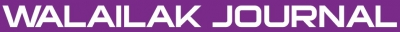A General Family of Fifth-Order Methods for Finding Simple Roots of Nonlinear Equations

Abstract

In this paper, a new fifth-order family of methods free from second derivative is obtained. The new iterative family contains the King’s, which is one of the most well-known family of methods for solving nonlinear equations, and some other known methods as its particular case. To illustrate the efficiency and performance of proposed family, several numerical examples are presented. Numerical results illustrate better efficiency and performance of the presented methods in comparison with the other compared fifth-order methods. Therefore, the proposed family can be effectively used for solving nonlinear equations.

Keywords

Iterative methods, Simple-root of nonlinear equations, Newton’s method

PDF

References

F Traub. Iterative Methods for the Solution of Equations. Prentice Hall, New Jersey, 1964.

C Chun. Some variants of King’s Fourth-order family of methods for nonlinear equations. Appl. Math. Comput. 2007; 190, 57-62.

AM Ostrowski. Solution of Equations in Euclidean and Banach Space. Academic Press, New York, 1973.

J Biazar and B Ghanbari. A new third-order family of nonlinear solvers for multiple roots. Comput. Math. .Appl. 2010; 59, 3315-9.

YM Ham and C Chun. A fifth-order iterative method for solving nonlinear equations. Appl. Math. Comput. 2007; 194, 287-90.

L Fang, L Sun and G He. An efficient newton-type method with fifth-order convergence for solving nonlinear equations. Comp. Appl. Math. 2008; 27, 269-74.

L Fang and G He. Some modifications of Newton’s method with higher-order convergence for solving nonlinear equations. J. Comput. Appl. Math. 2009; 228, 296-303.

YM Ham, C Chun and S Lee. Some higher-order modifications of Newton’s method for solving nonlinear equations. J. Comput. Appl. Math. 2008; 222, 477-86.

M Grau and JL Díaz-Barrero. An improvement of the Euler-Chebyshev iterative method. J. Math. Anal. Appl. 2006; 315, 1-7.

J Kou and Y Li. The improvements of Chebyshev-Halley methods with fifth-order convergence. Appl. Math. Comput. 2007; 188, 143-7.

W Gautschi. Numerical Analysis: An Introduction. Birkhäuser, 1997.

Refbacks

• There are currently no refbacks.Online ISSN: 2228-835X

http://wjst.wu.ac.th

Last updated: 17 May 2019